Courses

# Multilane Highways (Part - 2) Civil Engineering (CE) Notes | EduRev

## Civil Engineering (CE) : Multilane Highways (Part - 2) Civil Engineering (CE) Notes | EduRev

The document Multilane Highways (Part - 2) Civil Engineering (CE) Notes | EduRev is a part of Civil Engineering (CE) category.
All you need of Civil Engineering (CE) at this link: Civil Engineering (CE)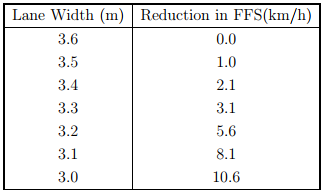Table 23:4: Adjustment for lateral clearance(Source: HCM, 2000)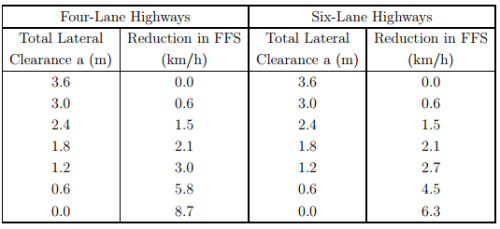Table 23:5: Adjustment to free flow speed for median type(Source: HCM, 2000)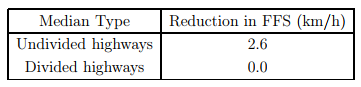Table 23:6: Adjustment to free flow speed for Access-point density(Source: HCM, 2000)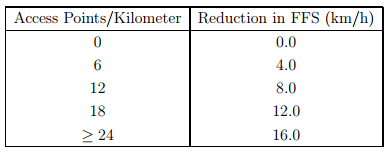(intersections and driveways) on the right side of the roadway in the direction of travel being studied by the length of the segment in kilometers. The adjustment factor for access-point density is given in Table 6. Thus the free flow speed can be computed using equation 1 and applying all the adjustment factors.

Determination of Flow Rate

The next step in the determination of the LOS is the computation of the peak hour factor. The fifteen minute passenger-car equivalent flow rate (pc/h/ln), is determined by using following formula:

where, vp is the 15-min passenger-car equivalent flow rate (pc/h/ln), V is the hourly volume (veh/h), PHF is the peak-hour factor, N is the number of lanes, fHV is the heavy-vehicle adjustment factor, and fp is the driver population factor. PHF represents the variation in traffic flow within an hour. Observations of traffic flow consistently indicate that the flow rates found in the peak 15-min period within an hour are not sustained throughout the entire hour. The PHFs for multilane highways have been observed to be in the range of 0.75 to 0.95. Lower values are typical of rural or off-peak conditions, whereas higher factors are typical of urban and suburban peak-hour conditions. Where local data are not available, 0.88 is a reasonable estimate of the PHF for rural multilane highways and 0.92 for suburban facilities. Besides that, the presence of heavy vehicles in the traffic stream decreases the FFS because base conditions allow a traffic stream of passenger cars only. Therefore, traffic volumes must be adjusted to reflect an equivalent flow rate expressed in passenger cars per hour per lane (pc/h/ln). This is accomplished by applying the heavy-vehicle factor (fHV ). Once values for ET and ER have

Table 23:7: Passenger-car equivalent on extended general highway segments(Source: HCM, 2000)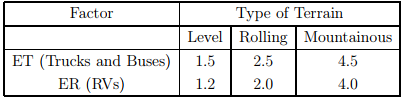been determined, the adjustment factors for heavy vehicles are applied as follows: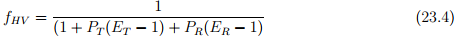where, ET and ER are the equivalents for trucks and buses and for recreational vehicles (RVs), respectively, PT and PR are the proportion of trucks and buses, and RVs, respectively, in the traffic stream (expressed as a decimal fraction), fHV is the adjustment factor for heavy vehicles. Adjustment for the presence of heavy vehicles in traffic stream applies for three types of vehicles: trucks, buses and recreational vehicles (RVs). Trucks cover a wide range of vehicles, from lightly loaded vans and panel trucks to the most heavily loaded coal, timber, and gravel haulers. An individual truck’s operational characteristics vary based on the weight of its load and its engine performance. RVs also include a broad range: campers, self-propelled and towed; motor homes; and passenger cars or small trucks towing a variety of recreational equipment, such as boats, snowmobiles, and motorcycle trailers. There is no evidence to indicate any distinct differences between buses and trucks on multilane highways, and thus the total population is combined.

Determination of Level of Service The level of service on a multilane highway can be determined directly from Fig. 23:12 or Table2 based on the free-flow speed (FFS) and the service flow rate (vp) in pc/h/ln. The procedure as follows:

1. Define a segment on the highway as appropriate. The following conditions help to define the segmenting of the highway,

• Change in median treatment

• Change in grade of 2% or more or a constant upgrade over 1220 m

• Change in the number of travel lanes

• The presence of a traffic signal

• A significant change in the density of access points

• Different speed limits

• The presence of bottleneck condition In general, the minimum length of study section should be 760 m, and the limits should be no closer than 0.4 km from a signalized intersection.

2. On the basis of the measured or estimated free-flow speed on a highway segment, an appropriate speed-flow curve of the same as the typical curves is drawn.

3. Locate the point on the horizontal axis corresponding to the appropriate flow rate (vp) in pc/hr/ln and draw a vertical line.

4. Read up the FFS curve identified in step 2 and determine the average travel speed at the point of intersection.

5. Determine the level of service on the basis of density region in which this point is located. Density of flow can be computed as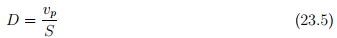where, D is the density (pc/km/ln), vp is the flow rate (pc/h/ln), and S is the average passenger-car travel speed (km/h). The level of service can also be determined by comparing the computed density with the density ranges shown in table given by HCM. To use the procedures for a design, a forecast of future traffic volumes has to be made and the general geometric and traffic control conditions, such as speed limits, must be estimated. With these data and a threshold level of service, an estimate of the number of lanes required for each direction of travel can be determined.

Numerical example 1

A segment of undivided four-lane highway on level terrain has field-measured FFS 74.0-km/h, lane width 3.4-m, peak-hour volume 1,900-veh/h, 13 percent trucks and buses, 2 percent RVs, and 0.90 PHF. What is the peak-hour LOS, speed, and density for the level terrain portion of the highway?

Solution The solution steps are given below:

1. Data given: Level terrain, field measured FFS = 74 km/h, lane width is 3.4 m, peakhour volume = 1900 veh/h, percent trucks and buses pt = 0.13, percent RVs PR = 0.02, and PHF=0.90.

2. Determination of flow rate(Vp): LOS can be calculated by knowing flow rate and free flow speed. Flow rate (Vp) is calculated from the equation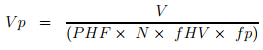Since fHV is unknown it is calculated from the equation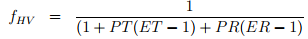where, ET and ER are passenger-car equivalents for trucks and buses and for recreational vehicles (RVs) respectively PT and PR are proportion of trucks and buses, and RVs, respectively, in the traffic stream (expressed as a decimal fraction)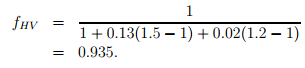Assume no RVs, since none is indicated.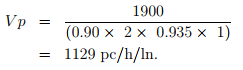3. Determination of free flow speed(S): In this example the free flow speed (FFS) measured at the field is given and hence no need to compute free flow speed by indirect method. Therefore, FFS = S = 74.0km/h.

4. Determination of density(D): The density of flow is computed from the equation D = V p/S = 15.3

5. Determination of LOS: LOS determined from the speed-flow diagram. LOS = C.

Numerical example 2

A segment of an east-west five-lane highway with two travel lanes in each direction separated by a two-way left-turn lane (TWLTL) on a level terrain has- 83.0-km/h 85th-percentile speed ,3.6-m lane width, 1,500-veh/h peak-hour volume, 6 % trucks and buses, 8 access points/km (WB), 6 access points/km (EB), 0.90 PHF, 3.6-m and greater lateral clearance for westbound and eastbound. What is the LOS of the highway on level terrain during the peak hour?

Solution The solution steps are enumerate below:

1. Data given: Level terrain, 85th-percentile speed is 83.0 km/h , lane width is 3.6 m, peakhour volume, v=1500 veh/h percent of trucks and buses PT=0.06, 8 access points/km in WB, 6 access points/km in EB, PHF = 0.90, and lateral clearance for westbound and eastbound is more than 3.6 m.

2. Determination of flow rate(VP): LOS can be calculated by knowing flow rate and free flow speed. Flow rate (Vp) is calculated from the equation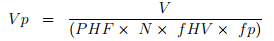where, Vp = 15-min passenger-car equivalent flow rate (pc/h/ln), V = hourly volume (veh/h), PHF = peak-hour factor, N = number of lanes, fHV = heavy-vehicle adjustment factor, and fp = driver population factor Since fHV is unknown it is calculated from the equation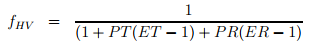where, ET and ER = passenger-car equivalents for trucks and buses and for recreational vehicles (RVs), respectively PT and PR = proportion of trucks and buses, and RVs, respectively, in the traffic stream (expressed as a decimal fraction) Assume no RVs, since none is indicated.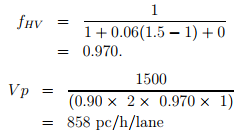3. Determination of free flow speed(S): BFFS is approximately equal to 62.4 km/h when the 85 th percentile speed is 64 km/h, and it is 91.2 km/h when the 85 th percentile speed is 96 km/h and the in between speed values is found out by interpolation. Hence, BFFS = 80 km/h. Now, compute east bound and west bound free-flow speeds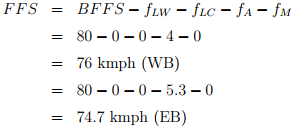4. Determination of LOS: LOS determined from the speed-flow diagram. LOS = C (for EB) LOS = C (for WB)

Numerical example 3

A 10 km long 4 lane undivided multilane highway in a suburban area has a segment 1 km long with a 3% upgrade and a segment 1 km long with a 3% downgrade. The section has a volume of 1900 vehicles/hr in each direction with 13% trucks and buses and 2% recreational vehicles. The 85 th percentile speed of passenger car is 80 km/hr on upgrade and 86km/hr on downgrade. There are total of 12 access points on both sides of the roadway. The lane width is 3.6 m, PHF is 0.90 and having a 3m lateral clearance. Determine the LOS of the highway section (upgrade and downgrade) during the peak hour? From HCM, For a 3% upgrade and 1 km length( ET=1.5 , ER=3) For a 3% downgrade and 1 km length( ET=1.5 , ER=1.2 )

Solution 1. Data given: 3%upgrade and 3%downgrade No of lanes = 4, N = 4, 80.0 km/h 85thpercentile speed for upgrade, 86 km/h 85t h-percentile speed for downgrade, 3.6-m lane width, 1,900-veh/h peak-hour volume, (V =1900 veh/h) 13 % trucks and buses, (PT =0.13) 2 % Recreational vehicles, ( Pr=0.02 ) 12 access points/km, PHF = 0.90 lateral clearance = 3 m

2. Determination of flow rate(VP): LOS can be calculated by knowing flow rate and free flow speed.

For upgrade: Flow rate is calculated from the equation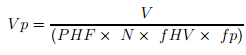where, Vp = 15-min passenger-car equivalent flow rate (pc/h/ln), V = hourly volume (veh/h), PHF = peak-hour factor, N = number of lanes, fHV = heavy-vehicle adjustment factor, and fp = driver population factor Since fHV is unknown it is calculated from the equation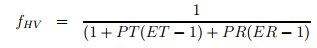where, ET and ER = passenger-car equivalents for trucks and buses and for recreational vehicles (RVs), respectively PT and PR = proportion of trucks and buses, and RVs, respectively, in the traffic stream (expressed as a decimal fraction) Assume no RVs, since none is indicated.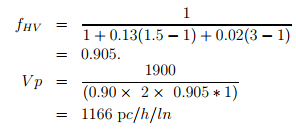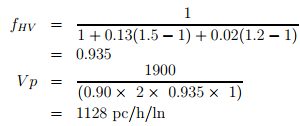3. Determination of free flow speed(S): For upgrade: BFFS is approximately equal to 62.4 km/h when the 85 th percentile speed is 64 km/h, and it is 91.2 km/h when the 85 th percentile speed is 96 km/h and the in between speed values is found out by interpolation. Hence for 86 km/hr 85th percentile speed from interpolation we get, BFFS = 77.0 km/h Now, Compute east bound and west bound free-flow speeds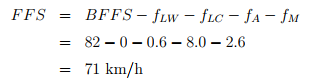For downgrade: BFFS is approximately equal to 62.4 km/h when the 85 th percentile speed is 64 km/h, and it is 91.2 km/h when the 85 th percentile speed is 96 km/h and the in between speed values is found out by interpolation. Hence for 86 km/hr 85th percentile speed from interpolation we get, BFFS= 82.0 km/h Now, Compute the free-flow speed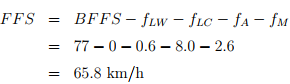Conclusion

This chapter helps to determine the level of service and capacity for a given road segment. In the first part we studied highways in general there classification and characteristics which gives the overall idea of multilane highways. Then we studied determination of capacity for multilane highway which is again a very important parameter used to determine the level of service, then we studied the concept of level of service and procedure to determine level of service. Also by using its applications, number of lanes required (N), and flow rate achievable (vp), Performance measures related to density (D) and speed (S) can also be determined.

4. Determination of LOS LOS determined from the speed-flow diagram. LOS = D (for upgrade) LOS = D (for downgrade)

Offer running on EduRev: Apply code STAYHOME200 to get INR 200 off on our premium plan EduRev Infinity!

,

,

,

,

,

,

,

,

,

,

,

,

,

,

,

,

,

,

,

,

,

;